# Log

if
${\mathrm{log}}_{4}x+{\mathrm{log}}_{8}x={\mathrm{log}}_{b}x$
, what is b?

Correct result:

b =  2.3

#### Solution:

$b={4}^{\frac{1}{1+\frac{log\left(4\right)}{log\left(8\right)}}}=2.3$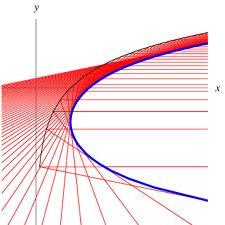We would be pleased if you find an error in the word problem, spelling mistakes, or inaccuracies and send it to us. Thank you!## Next similar math problems:

• Intercept with axisF(x)=log(x+4)-2, what is the x intercept
• Exponential equationIn the set R solve the equation: ?
• LogarithmDetermine the number whose decimal logarithm is -3.8.
• Exponential equationFind x, if 625 ^ x = 5 The equation is exponential because the unknown is in the exponential power of 625
• Exponential equationSolve for x: (4^x):0,5=2/64.
• SequenceCalculate what member of the sequence specified by ? has value 86.
• Coordinate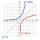Determine missing coordinate of the point M [x, 120] of the graph of the function f bv rule: y = 5x
• DecibelBy what percentage does the sound intensity increase if the sound intensity level increases by 1 dB?
• The city 3The city has 22,000 residents. How long it is expected to have 25,000 residents if the average annual population growth is 1.4%?
• If you 4If you deposit $2500 in an account paying 11% annual interest compounded quarterly, how long until there is the$4500 in the account?
• If you 2If you deposit $4000 into an account paying 9% annual interest compounded monthly, how long until there is$10000 in the account?
• DemographicsThe population grew in the city in 10 years from 30000 to 34000. What is the average annual percentage increase of population?
• Exp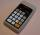If ?, then n is:
• Sum-log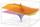The sum of two numbers is 32, the sum of their logarithms (base 10) is 2.2. Determine these numbers.
• Exponential equationSolve exponential equation (in real numbers): 98x-2=9
• Log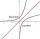Calculate value of expression log |3 +7i +5i2| .
• Logarithmic equation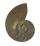Solve equation: log33(3x + 21) = 0